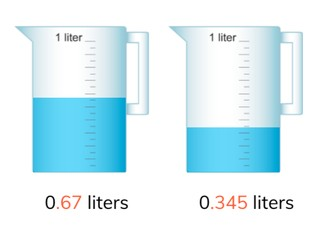Determine difference between decimal numbers with 1, 2, and 3 decimals

# Determine difference between decimal numbers with 1, 2, and 3 decimals

No account needed.8,000 schools use Gynzy92,000 teachers use Gynzy1,600,000 students use Gynzy

## General

Students learn to determine the difference between decimal numbers with 1, 2, and 3 decimals.

6.NS.B.3

## Relevance

It is important to be able to determine the difference, so you can tell which numbers are more or less than another. You can use this to determine how much is in a measuring cup.

## Introduction

The interactive whiteboard shows a measuring scale. Calculate how many pounds still need to be added. The students do this with weights with one, two and three decimals. Ask students to explain their strategy.

## Development

Explain to students that if you want to determine the difference between two numbers, that you are looking to see how many more, or how many less one number is than the other. When the decimal numbers have different amounts of numbers after the decimal point you can add a zero or zeros to the hundredths and thousandths places. Explain to students that there are two ways to determine difference. The first is to use a number line to determine the difference between two numbers, by counting up from the smaller number to the larger number. Show this in three steps. First you jump from the decimal number to the next whole number. Then you jump to the next whole number. Finally you jump to the decimal number again. The second way to determine difference is to make a subtraction problem using the two decimal numbers. For example you can use the numbers 56.729 and 24.3 to make the subtraction problem 56.729 - 24.300 to determine the difference. Remind students that this method does not work if the numbers after the decimal point of the second number (subtrahend) are greater than those of the first number (minuend). Ask students to solve some problems and to explain their strategy. Next discuss how to solve a decimal story problem. Remind students to first determine what kind of math problem they will make, then to determine which numbers are important, to say or write the problem, and finally to solve the problem. Solve one story problem as a class and then as students to solve the next decimal story problem individually or in pairs.

Check that students understand subtracting decimal numbers with 1, 2, and 3 decimal places by asking the following questions:
- What are two ways in which to determine the difference between two decimal numbers?
- Which subtraction problem can you form with the decimal numbers 893.2 and 368.35 to determine the difference?
- What are the steps to solving a story problem?

## Guided practice

Students are asked to find the difference between two given decimal numbers. They are also asked to complete a table which contains a number of subtraction problems and to solve a story problem. Ask students to think about what strategy they use to solve the subtraction problems.

## Closing

Remind the students of the learning goal and its importance. On the interactive whiteboard, a scale is shown with two different weights. Students must find the difference between the two given weights. Ask students to explain their strategy, and you can choose to have a student come up to solve the subtraction problem on the interactive whiteboard. Encourage students to add a zero or zeros to the hundredths and thousandths places to make both numbers have the same number of digits after the decimal point.

## Teaching tips

Students who have difficulty with this learning goal can be supported by making use of the number line. Splitting the subtrahend and making those smaller jumps on the number line helps make the progression more visible.

## Instruction materials

...Instruction materials text...

### The online teaching platform for interactive whiteboards and displays in schools

• Save time building lessons

• Manage the classroom more efficiently

• Increase student engagement